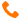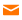Group Services: Technology Consulting+91-9999-283-283/9540-283-283info@sisoft.in

# Java Multi-Threading Example

## MultiThreading Example (Extending Thread, Implementing Runnable)``` public class MultiThreadingExample extends Thread { public static void main(String[] args) { // Start Thread class myThread mt1 = new myThread(); mt1.start(); // start Runnable Class myRunnable mr1 = new myRunnable(); Thread tr1 = new Thread(mr1); tr1.start(); for (int i=0; i<100; i++) { System.out.println(Thread.currentThread().getName()+":"+i); } } } class myThread extends Thread{ @Override public void run() { // TODO Auto-generated method stub super.run(); for (int i=0; i<100; i++) { System.out.println(Thread.currentThread().getName()+":"+i); } } } class myRunnable implements Runnable{ @Override public void run() { // TODO Auto-generated method stub for (int i=0; i<100; i++) { System.out.println(Thread.currentThread().getName()+":"+i); } } } ```

## Thread Synchronization (synchornize)``` class NeedForSynchronizationDemo { public static void main (String [] args) { FinTrans ft = new FinTrans (); TransThread tt1 = new TransThread (ft, "Deposit Thread"); TransThread tt2 = new TransThread (ft, "Withdrawal Thread"); tt1.start (); tt2.start (); } } class FinTrans { public static String transName; public static double amount; } class TransThread extends Thread { private FinTrans ft; TransThread (FinTrans ft, String name) { super (name); // Save thread's name this.ft = ft; // Save reference to financial transaction object } public void run () { for (int i = 0; i < 100; i++) { if (getName ().equals ("Deposit Thread")) { // Start of deposit thread's critical code section ft.transName = "Deposit"; try { Thread.sleep ((int) (Math.random () * 1000)); } catch (InterruptedException e) { } ft.amount = 2000.0; System.out.println (ft.transName + " " + ft.amount); // End of deposit thread's critical code section } else { // Start of withdrawal thread's critical code section ft.transName = "Withdrawal"; try { Thread.sleep ((int) (Math.random () * 1000)); } catch (InterruptedException e) { } ft.amount = 250.0; System.out.println (ft.transName + " " + ft.amount); // End of withdrawal thread's critical code section } } } } package in.sisoft; // Example to show case synchronized at instance level method class AccountHandling implements Runnable{ int b1 ; int sleeptime ; BankAccount b2 ; AccountHandling(){ b2 = new BankAccount() ; b2.setBalace(4000.0f); } public void run(){ System.out.println("thread is running..."); b2.sleeptime=sleeptime; try { b2.withdraw(3000); } catch (InterruptedException e) { // TODO Auto-generated catch block e.printStackTrace(); } } public static void main(String[]args){ AccountHandling a=new AccountHandling(); a.b1=4 ; a.sleeptime= 200 ; Thread t1 =new Thread(a); a.sleeptime=300 ; Thread t2 =new Thread(a); t1.start(); t2.start(); } } class BankAccount{ public String getName() { return name; } public void setName(String name) { this.name = name; } public Float getBalace() { return balace; } public void setBalace(Float balace) { this.balace = balace; } String name ; Float balace ; public int sleeptime ; // Remove synchronized in below method and check the output. synchronized boolean withdraw(float amt) throws InterruptedException{ float bal = getBalace(); Thread.sleep(sleeptime); if (bal > amt){ bal = getBalace() - amt ; setBalace(bal); System.out.println("Amount will be withdrawn:"+ bal); return true ; } else { System.out.println("Insufficient balance"); return false ; } } } ```

## Executor Framework``` import java.util.concurrent.ExecutorService; import java.util.concurrent.Executors; public class ServiceExecutor { public static void main(String[] args) { // TODO Auto-generated method stub ExecutorService es = Executors.newFixedThreadPool(6); for (int i=0; i<10; i++){ es.execute(new Runnable() { public void run() { try{ Thread t1 = Thread.currentThread(); System.out.println("Asynchronous task:"+t1.getName()); Thread.sleep(2000); System.out.println("End Thread :"+Thread.activeCount()); }catch (Exception e) {} ; } }); } es.shutdown(); } } ```